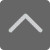# 小学口算题大全：二年级100以内加减法口算题（三）

2018-11-02 16:32:40 　来源：网络 文章作者：网络
 免费下载 3个G小报手抄报电子版 小学1-5年级上册期末复习资料 [ 戳我免费下载 ]

45+33+7= 57+28= 73+11= 86+8+2=

36+22= 22+19= 89+1+9= 57-2=

96-70= 56+33+7= 48-39= 55+27=

86+8+2= 66-6= 25+23= 2+80+10=

52+12= 22-22= 62+27+3= 14+27=

66+13= 69+20+10= 49-12= 72+5+5=加入QQ群，与更多家长交流经验！！！
 上海小学交流群：639215153 上海初中交流群：551802726 上海高中交流群：591337342 上海幼升小交流群：772707735